What are the different properties of trapezium?

• Difficulty Level : Expert
• Last Updated : 02 Nov, 2021

Mensuration is the branch of mathematics or a subject of geometry that deals with geometric figures and parameters like length, volume, area, shape, surface area, size, region, etc. In simple words, when we talk about the length, area, volume of specific shapes or different parameters of geometric figures then it is called Mensuration.

In Mensuration shapes exist in 2 dimensions or 3 dimensions shape.

Mensuration Formulas

• Square: Area = a2  , Perimeter = 4a
• Rectangle: Area = L x B  , Perimeter = 2 ( L + B )
• Circle: Area = π r  , Perimeter =2πr
• Isosceles Triangle: Area = 1/2 x b x h   , Perimeter = a +b + 2c
• Equilateral triangle: Area = ( √3/4 ) x a2   , Perimeter = 3a
• Right Angle Triangle: Area = 1/2 x b x h   , Perimeter = b + hypotenuse + h
• Rhombus: Area = 1/2 x d1 x d2  , Perimeter = 4 x side
• Parallelogram: Area = b x h  , Perimeter = 2 ( l + b )
• Trapezium: Area = 1/2 h ( a + c)   , Perimeter = a + b + c + d

What is a Trapezium?

A trapezium is a closed shaped two-dimensional quadrilateral having a pair of parallel opposite sides. The parallel sides of a trapezium are called bases and the non-parallel sides of a trapezium are called legs. Trapezium has four sides and four corners. Parallelogram is also called a trapezoid with two parallel sides.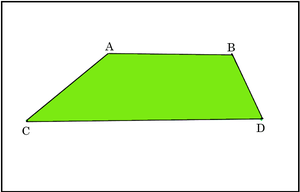Figure – 1

In figure 1, AB and CD are base of trapezium and AC and BD are the legs of the trapezium.

Types of Trapezium

Based on the sides and the angles, trapezium are of three types:

• Isosceles Trapezium
• Scalene Trapezium
• Right Trapezium

Isosceles Trapezium

The trapezium which has an equal length of legs is called an isosceles trapezium.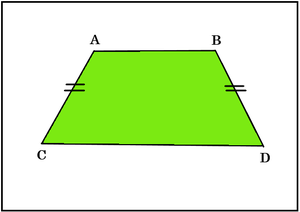Figure – 2

As you see in figure 2, AC and BD are of equal lengths.

Scalene Trapezium

A trapezium with all the sides and angles that are not equal is called a scalene trapezium.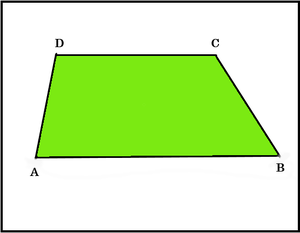Figure – 3

Right Trapezium

A trapezium that has right angle in a pair, adjacent to each other is known as right trapezium.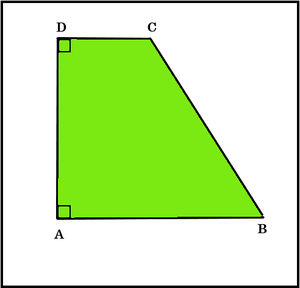Figure – 4

Properties of Trapezium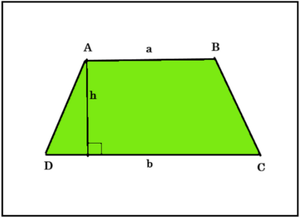Figure – 5

• In trapezium, bases are parallel to each other.

Example – The sides AB and CD are parallel to each other, shown in figure 5.

• The adjacent interior angles in a trapezium sum up to be 180°.

Example – There are two pairs of co-interior angles. One pair is ∠A and ∠D whereas the other pair is ∠B and ∠C. The sum of each pair of co-interior angles is 180°.

• The sum of all the interior angles in a trapezium is always 360°.

Example – In figure 5, ∠A+∠D is 180°  and ∠B+∠C is 180°. Therefore ∠A+∠D +∠B+∠C = 360°.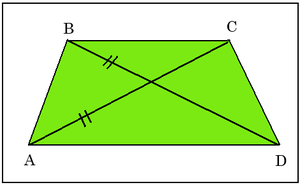Figure – 6

• The length of both the diagonals in the trapezium is equal.

Example – In figure 6, Diagonal AC = Diagonal BD.

• In trapezium both the diagonals intersect each other.

Example – AC and BD are intersecting with each other as shown in figure 6.

• Trapezium has exactly one pair of opposite sides that are parallel.

Area and Perimeter of Trapezium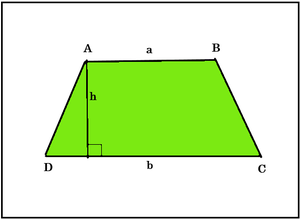Figure – 7

In figure 7, the trapezium having length of parallel sides a, b units respectively and the altitude h.

We can find the area of trapezium by calculating the average of bases and multiplying its result to the altitude.

Hence,

Area of trapezium = ((AB + DC)/2) × AM =((a +b)/2) × h

where AB and CD are the bases of trapezium and AM is the altitude as shown in figure.

The perimeter of a trapezium is given by calculating the sum of all its sides.

Hence,

Perimeter of trapezium = AB + BC + CD + AD

where AB, BC, CD and AD are the sides of the trapezium.

Sample Questions

Question 1. What is the shape of a trapezium?

A trapezium is a two-dimensional 2D closed shape having four straight sides, with one pair of parallel sides.

Question 2. A trapezium has parallel sides of length 15 cm and 11 cm, and non-parallel sides of length 5 cm each. Calculate the perimeter of the trapezium?

It is a Isosceles Trapezium because it is clearly mentioned that non parallel sides of length 5 cm each are equal. According to the isosceles trapezium if two non-parallel sides of the trapezium are of equal length then it is known as isosceles trapezium.

Given, a=15 cm, b=11 cm and c= 5 cm

We get,

Perimeter = a+b+2c

P = 15+11+2(5)

P = 15+11+10

P = 36 cm

Question 3. Find the perimeter of a trapezium whose sides are 12cm, 14cm, 16cm and 18cm.

As we know that the perimeter of a trapezium is given by calculating the sum of all its sides.

P = Sum of all the sides

P = 12 + 14 + 16 + 18

P = 60 cm

Hence, the perimeter of trapezium is 60 cm.

Question 4. Find the area of the Trapezium, in which the sum of the parallel sides is 60cm, and its height is 10cm.

Given, the sum of parallel sides 60cm and the height, h =10cm

We know that,

Area of a trapezium, A = 1/2 × Sum of parallel sides × distance between the parallel sides

By substituting the given values,

A =1/2×60×10

A = 30×10

A = 300 cm2.

Therefore, the area of Trapezium =300cm2.

Question 5. What are the 5 properties of a trapezium?

The 5 properties of a Trapezium are:

1. In trapezium, bases are parallel to each other.

2. A trapezium has supplementary adjacent angles.

3. Only one pair of opposite sides are parallel.

4. The sum of all the interior angles in a trapezium is always 360°.

5. The line that joins the mid-point of the non-parallel sides is always parallel to the bases.

My Personal Notes arrow_drop_up